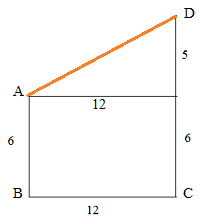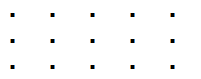# TCS NQT Ninja and Digital placement questions - 20

1. problemsolvingproblemsolvingprob . . . . . . Find the 2015th term in the series?
Sol.
Problemsolving = 14 letter word. So divide 2015 by 14 and find the remainder. Here remainder is 13. so 13th letter in problemsolving is 'n'

2. 4 men can check exam papers in 8 days working 5 hours regularly. What is the total hours when 2 men will check the double of the papers in 20 days?
Sol.
Let a man can do 1 unit of work in 1 hour.
Total units of work = 4 x 8 x 5 = 160 units.
Now work = 2 x 160 = 320 units.
Now 2 men work for 20 days. Let in x hours they have to work per day.
Now total work = 2×x×20 = 40 x
40x = 320  So x = 320/40 = 8 hours.

3. X = 101102103104105106107......146147148149150  (From numbers 101-150).  Find out the remainder when this number is divided by 9.
Sol:
The divisibility rule for 9 is sum of the digits is to be divisible by 9.  So
We calculate separately, sum of the digits in hundreds place, tenths place, and units place.
Sum of the digits in hundreds place: 1 x 50 = 50
Sum of the digits in tenths place : 0 x 9 + 1 x 10 + 2 x 10 + 3 x 10 + 4 x 10 + 5 x 1 = 105
Sum of the digits in units place : (1 + 2 + 3 + ...+ 9) x 5 = 225
So total = 380
So remainder = 380 / 9 = 2

4. A number is 101102103104...150. As 101 102 103 103.... 150. What is reminder when divided by 3?
Sol. Divisibility rule for 3 also same as 9. so from the above discussion sum of the digits = 380 and remainder = 380/3 = 2.
5. In 4 years, Raj's father age twice as raj, Two years ago, Raj's mother's age twice as raj. If Raj is 32yrs old in eight yrs from now, what is the age of Raj's mother and father?
Sol. Raj present age = 32 - 8 = 24.
After 4 years Raj's age is 28. and Raj's fathers age is 28 x 2 = 56, and his present age is 52.
Two years ago, Raj's age is 22. and his mother's age is 22 x 2 = 44. His mother's present age = 46

6. 7^1+7^2+7^3+.......+7^205. Find out how many numbers present which unit place contain 3?
Sol. Units digits of first 4 terms are 7, 9, 3, 1. and this pattern repeats. So for every 4 terms we get one term with 3 in its unit digit. So there are total of 205/4 = 51 sets and each set contains one terms with 3 in its unit digit.
Ans is 51.

7. In paper A, one student got 18 out of 70 and in paper B he got 14 out of 30. In which paper he did fare well?
Sol. Find the percentages. Paper A = 18/70 x 100 = 25.7
Paper B = 14/30 x 100 = 46.6
8. Find the total no of divisors of 1728 (including 1 and 1728)
The number of factors or divisors of a number ${\rm{N = }}{{\rm{a}}^{\rm{p}}}{\rm{.}}{{\rm{b}}^{\rm{q}}}{\rm{.}}{{\rm{c}}^{\rm{r}}}...$ = (p+1).(q+1).(r+1)... where a, b, c ... prime numbers.
1728 = ${2^6} \times {3^3}$
So total number of divisors = (6 + 1).(3 + 1) = 28

9. The sum of two numbers is 45. Sum of their quotient and reciprocal is 2.05, Find the product of the numbers.
Sol: Let a, b be the numbers.
a + b = 45
$\displaystyle\frac{a}{b} + \frac{b}{a}$ = 2.05
$\Rightarrow \displaystyle\frac{{{a^2} + {b^2}}}{{ab}}$ = 2.05
$\Rightarrow \displaystyle\frac{{{{\left( {a + b} \right)}^2} - 2ab}}{{ab}} = 2.05$
$\Rightarrow {\left( {a + b} \right)^2}$ = 2.05ab + 2ab = 4.05ab
$\Rightarrow$ ab = $\frac{{{{45}^2}}}{{4.05}}$ = 500

10. A number is divided by 406 leaves remainder 115 , What will be the reminder when it will be divided by 29?
Sol. Let the number be N.
So N = 406x + 115.
Now divide this number by 29. As 406 is exactly divisible by 29, we have to divide 115 by 29 and find the remainder. So remainder = 28

11. (p/q-q/p)=21/10. Then find 4p/q+4q/p?
sol.
Let p/q = a, then (a - 1/a) = 21/10
$\Rightarrow {a^2} - 1 = a.\displaystyle\frac{{21}}{{10}}$
$\Rightarrow 10{a^2} - 21a - 10$ = 0
Roots of the equation = $\displaystyle\frac{{ - b \pm \sqrt {{b^2} - 4ac} }}{{2a}}$
a = $\displaystyle\frac{{ 21 \pm \sqrt {441 + 400} }}{{20}}$
a = $\displaystyle\frac{{21 \pm 29}}{{20}}$ = 5/2 or -2/5
For a = 5/2, 4p/q+4q/p = 58/5
For a = -2/5, 4p/q+4q/p = -58/5

12. Two vertical ladders length of 6 m and 11 m are kept vertically at a distance of 12 m. Find the top distance of both ladders?
Sol:So distance between the top points = AD = $\sqrt {{{12}^2} + {5^2}} = 13$
So in paper B he did well.

Updated:

13.Here is 15 dots.  If you select 3 dots randomly, what is the probability that 3 dots make a triangle?
a. 440/455
b. 434/455
c. 449/455
d. 438/455
Sol.I think there seem some problem with this question. Total ways of selecting 3 dots out of 15 is ${}^{15}{C_3}$ = 455 If 3 dots are collinear then triangle may not be formed. Now look at the above diagram.  If we select any 3 dots from the red lines they may not form a triangle. They are 3 x ${}^{5}{C_3}$ = 30.  If we select the three letters from blue lines, they may not form a triangle. They are in total 5 ways. Also there are 6 others lines which don't form a triangle.  Also another two orange lines. Total = 30 + 5 + 6 + 2 = 43.  So we can form a triangle in 455 - 43 = 412. So answer could be 412/455.

14.   In a series of numbers , the next number is formed by adding 1 to the sum of the previous numbers, and the 10th number is 1280.  Then what is the first number in the series? (series will be like this x , x+1, (x+(x+1))+1,....... )

a. 1
b. 4
c. 5
d. None of these
Sol.
The given series is x, x + 1, 2x + 2, 4x + 4 ......
If you observe the pattern here,  the coefficient of x + 1 is in the powers of 2. So 4th term has a power of 2, 5th term has a power of 3... 10th term has a power of 8. So tenth term would be ${2^8}$(x + 1)
= 256(x+1).
Given 256(x+1) = 1280
x = 4.

15. The number of multiples of 10 which are less than 1000, which can be written as a sum of four consecutive integers is

a. 50
b.100
c. 150
d. 216
Sol:
We can write 10 = 1 + 2 + 3 + 4.  So we have to find how many multiples of 10 can be written in this manner.
Let the first of the four numbers be n. So
n + (n+1) + (n+2) + (n+3) = 10k
4n + 6 = 10k
2n + 3 = 5k
n = $\displaystyle\frac{{5k - 3}}{2}$ = 2k – 1 + $\displaystyle\frac{{k – 1}}{2}$
So n is intezer for k = an odd number. So for k = 1, 3, 5, .... 99 we can write a number as a sum of four consecutive intezers.
So there are 50 numbers.

16. Mr. Bean chooses a number and he keeps on doubling the number followed by subtracting one from it, if he chooses 3 as initial number and he repeats the operation for 30 times then what is the final result?

a. (2^30) – 1
b. (2^30) – 2
c. (2^31) – 1
d. (2^31) – 2
Ans: No option
Sol:
Step 1: (3 x 2) - 1 = 5 ( 2^2 + 1)
Step 2: (5 x 2) - 1 = 9 (2^3 + 1)
Step 3: (9 x 2) - 1 = 17 (2^4 + 1)
Step 4: (17 x 2) - 1 = 33 (2^5 + 1)
So After 30 steps we have 2^31 + 1

17. Tony alone can paint a wall in 7 days and his friend Roy alone can paint the same wall in 9 days. In how many days they can paint the wall working together? Round off the answer to the nearest integer.

a. 3
b. 4
c. 5
d. 7
Sol. use formula (xy / x+y)
So nearest value for 3.93 = 4

18. In this question, A^B means A raised to the power B. Let f(X)=1+X+x^2+....x^6.  The remainder when f(X^7) is divided by f(X) is
a. 0
b. 6
c. 7
d. None of the other 3 choices.
Given that $f({x^7}) = 1 + {x^7} + {\left( {{x^7}} \right)^2}$ + ....+ ${\left( {{x^7}} \right)^6}$ = $1 + {x^7} + {x^{14}} + .... + {x^{42}}$
We will rewrite the above equation, $f({x^7}) = 1 + \left( {{x^7} - 1} \right) + \left( {{x^{14}} - 1} \right) +$... + $\left( {{x^{42}} - 1} \right) + 6$
We know that ${x^7} - 1 = (x - 1)({x^6} + {x^5} + ...1)$
($\because$ ${x^n} - {a^n}$ = $\left( {x - a} \right)$.$({x^{n - 1}} + {x^{n - 2}}.a +$${x^{n - 3}}.{a^2}..... + {a^{n - 1}}$ )
Now It is clear that ${x^7} - 1$ is exactly divisible by f(x).
Also ${x^{14}} - 1 = {\left( {{x^7}} \right)^2} - {1^2}$ and ${x^7} - 1$ is a factor of this expression.  ($\because {x^n} - {a^n}$ is always divisible by $x - a$
Similarly, we write ${x^{21}} - 1 = {\left( {{x^7}} \right)^3} - {1^3}$, ${x^{28}} - 1 = {\left( {{x^7}} \right)^4} - {1^4}$....